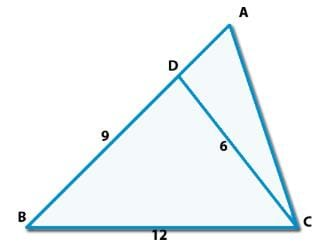Consider the triangle shown in the figure whe...

### Related TestConsider the triangle shown in the figure where BC = 12 cm, Db = 9 cm, CD = 6 cm and What is the ratio of the perimeter of the triangle ADC to that of the triangle BDC ?• a)
7:9
• b)
8:9
• c)
6:9
• d)
5:9
• e)
None of theseANUSHA MARRIHere, ∠ACB = c+180-(2c-b) = 180-(b+c)
So, We can say that Δ BCD and &delta ABC will be similar.
According to property of similarity,
AB/12 = 12/9
Hence,
AB = 16
AC/6 = 12/9
AC = 8
Hence, AD = 7 and AC = 8
Now,
Perimeter of Delta; ADC / Perimeter of &delta BDC,
= (6+7+8)/(9+6+12)
= 21/27 = 7/9.View courses related to this question Explore GMAT courses
 Explore GMAT coursesView courses related to this question1 Crore+ students have signed up on EduRev. Have you?

• ### In a triangle, one side is 6 Cm and another side is 9 Cm. which of the foll... more(Scan QR code)